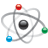## Triggering ADC with PDB in K66 microcontroller

cancel
Showing results for
Search instead for
Did you mean:

## Triggering ADC with PDB in K66 microcontroller

273 ViewsContributor I

Hello all,

I am trying to trigger ADC0 of the Teensy 3.6 with the PDB. I want 2 measurements per PWM period, so I have used FTM3 as a trigger input to PDB0 with 2 pretriggers. The delay of PDB0_CH0DLY0 is 0 while the delay of PDB0_CH0DLY1 is MOD/2.

This is all working correctly. However, I have a weird issue. ADC0 always makes 2 conversions for each trigger, so I get 4 measurements, 2 of which are redundant. To put it simply, ADC0_SC1A and ADC0_SC1B always convert together for each pretrigger.

I have the code of FTM3, PDB and ADC0 below respectively. [Note: MOD value is equal to 599 while Phi is equal to 200.]

FTM3:

void initFTM3(void) {
SIM_SCGC3    |= 0x02000000;

FTM3_SC      = 0x00;
FTM3_CONF    = 0xC0;
FTM3_FMS    = 0x00;
FTM3_MODE  |= 0x05;
FTM3_C0SC    = 0x28;
FTM3_C1SC    = 0x28;
FTM3_C2SC    = 0x28;
FTM3_C3SC    = 0x28;
FTM3_C4SC    = 0x68;
FTM3_C5SC    = 0x28;
FTM3_COMBINE  = 0x030303;
FTM3_COMBINE |= 0x1010;
FTM3_COMBINE |= 0x202020;
FTM3_DEADTIME = 0x00;
FTM3_CNT      = 0x00;
FTM3_CNTIN    = 0x00;
FTM3_MOD      = MOD;
FTM3_SYNC    = 0x02;
FTM3_SC      = 0x28;
PORTD_PCR0    = 0x400;
PORTD_PCR1    = 0x400;
PORTD_PCR2    = 0x400;
PORTD_PCR3    = 0x400;
PORTC_PCR8    = 0x300;
PORTC_PCR9    = 0x300;
FTM3_C0V      = (MOD+1)/2 - Phi;
FTM3_C1V      = (MOD+1)/2;
FTM3_C2V      = 0;
FTM3_C3V      = (MOD+1) - Phi;
FTM3_C4V      = ((MOD+1) - Phi)/2 - 8;
FTM3_C5V      = (2*(MOD+1) - Phi)/2 - 8;
FTM3_EXTTRIG |= 0x04;
asm("nop");
}

PDB:

void initPDB0(void) {
SIM_SCGC6    |= 0x400000;
PDB0_CNT      = 0;
PDB0_MOD      = MOD;
PDB0_CH0C1  |= 0x303;
PDB0_CH0DLY0  = 0;
PDB0_CH0DLY1  = (MOD+1)/2;
PDB0_SC      = 0xBA1;
}

ADC0:

void initADC0(void) {
SIM_SCGC6    |= 0x08000000;
SIM_SOPT7    = 0x00;
ADC0_CFG1    = 0x04;
ADC0_CFG2    = 0x04;
ADC0_SC2      = 0x40;
ADC0_SC3      = 0x00;
ADC0_SC1A    = 0x4C;
ADC0_SC1B    = 0x51;
NVIC_SET_PRIORITY(IRQ_ADC0, 128);
NVIC_CLEAR_PENDING(IRQ_ADC0) |= 1<<7;
NVIC_ISER1                  |= 1<<7;
NVIC_ENABLE_IRQ(IRQ_ADC0);
asm("cpsie i");
}

Below is the ISR of ADC0 to read measurements from the results registers ADC0_RA and ADC0_RB.

void adc0_isr(void) {
bool control = true;
if (ADC0_SC1A & 0xCC) {
V_analog = ADC0_RA;
V_o = VoutScale*V_analog;
operation();
control = !control;
}
if (ADC0_SC1B & 0xD1) {
V_analog = ADC0_RB;
V_in = VinScale*V_analog;
control = !control;
}
asm("nop");
}

The value of the variable control is always TRUE, which means it is toggled twice for each pretrigger which means ADC0_SC1A and ADC0_SC1B convert twice. I want just one conversion per pretrigger.

Does anyone know why this happens? Is there something I missed in the initialization or if I have a mistake in the code?

Thanks in advance.

Labels (3)

• ### Kinetis K Series MCUs

2 Replies
198 ViewsNXP TechSupport

Hi,

if (ADC0_SC1A & 0xCC) . This judgement may have some problems. It should be the
if (ADC0_SC1A & 0x80). Because you should judge the bit COCO of SCx. If you use 0xcc to &, then you will find if the conversion complete flag not being set, the judgement is also true. The judgement of SC1B has the same problem.

Have a great day,
TIC

-------------------------------------------------------------------------------
Note:
- If this post answers your question, please click the "Mark Correct" button. Thank you!

- We are following threads for 7 weeks after the last post, later replies are ignored
Please open a new thread and refer to the closed one, if you have a related question at a later point in time.
-------------------------------------------------------------------------------

198 ViewsContributor I

Hello,

thank you very much. This helped with the correct calling of the result registers.

However, I think two conversions are still happening when the PDB triggers the ADC the first time in the period.

To explain why I think it is:

1. I am triggering PDB at time FTM3_C4V = ((MOD+1) - Phi)/2; which is equal to (600-200)/2 = 200.
2. Pretrigger 1 delay PDB0_CH0DLY0 is equal 0.
3. Pretrigger 2 delay PDB0_CH0DLY1 is equal (MOD+1)/2 which is equal to 300.
4. I probe FTM3 counter register FTM3_CNT at the beginning of adc0_isr.
5. If I understand correctly, conversion time plus interrupt latency should equal around 30 counts.
6. So FTM3_CNT at adc0_isr should be around 230 and 530 for pretrigger 1 and 2 respectively.
7. However, what I get is 260 and 530 for pretrigger 1 and 2 respectively.
8. I think that the extra 30 counts in the first pretrigger is due to both conversions happening, while the second pretrigger value is correct.

Hope this explains what I am trying to say.

Regards.